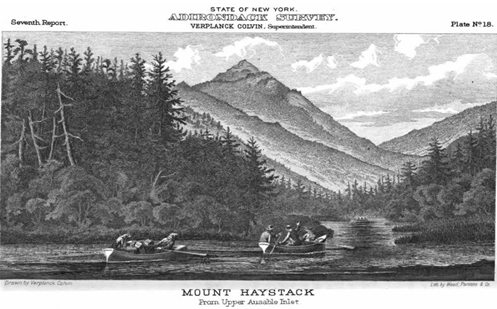Explore

Seeing mathematics in a ’real-world’ context helps students understand the relevance of the mathematics they are learning. This is a fascinating history of the application of trigonometry to surveying. There are interactive demonstrations of the techniques used.

Year level(s) Year 10
Audience Teacher
Purpose Teaching resource, Content knowledge
Keywords Angle, Length, Height, Tangent, Cosine, Sine, Trigonometry, Depression, Elevation

## Curriculum alignment

Curriculum connections Critical and creative thinking
Strand and focus Space
Topics Pythagoras and trigonometry
AC: Mathematics (V9.0) content descriptions
AC9M10M03

Solve practical problems applying Pythagoras' theorem and trigonometry of right-angled triangles, including problems involving direction and angles of elevation and depression

Numeracy progression Understanding geometric properties (P7)
Understanding units of measurement (P10)